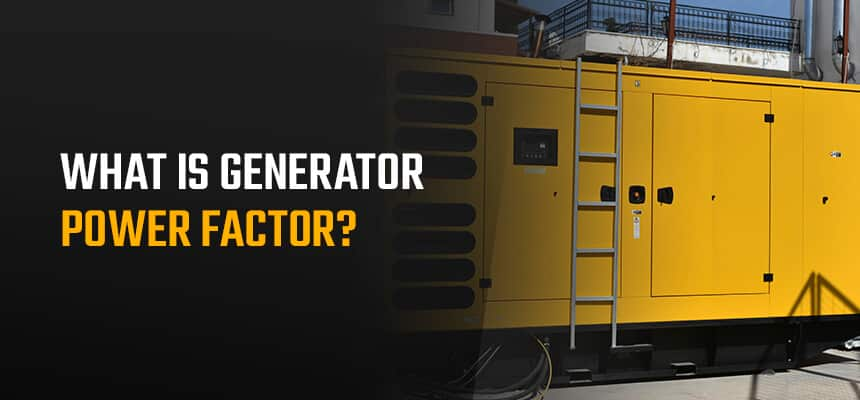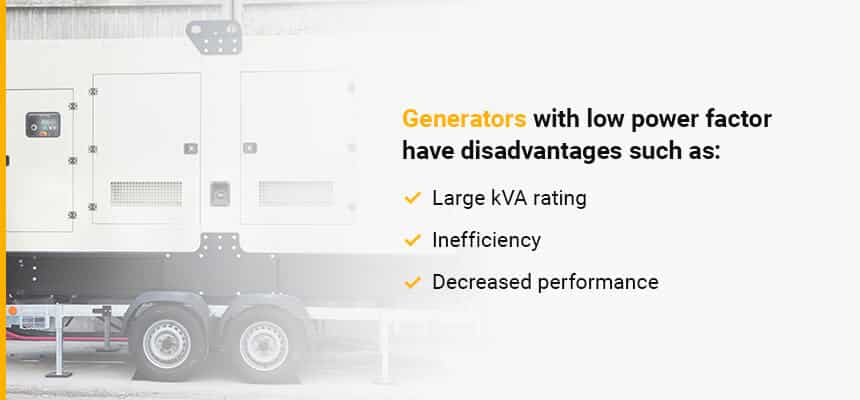## What is the Definition of Generator Power Factor?When you’re in the market for a generator or are looking to understand your current machine better, generator power factor is a crucial concept to understand. Power factor calculation looks at the details of how a generator runs so you can maximize productivity with the generator size you have and spot any issues early.

Different size generators have varying power factors that influence the loads they’re designed to handle. From definitions and measurement to the difference between apparent power and real power, learning more information about your generator is the first step toward more efficient operations.

## Generator Power Factor Explained

As you think about the best next steps for your commercial generator, you may be wondering, what does generator power factor mean? Generator power factor, or generator power rating, measures how efficiently a machine uses its energy. Typically expressed as a decimal or percentage, this value indicates the total current your generator can use to perform a certain job.

Ideally, all power a load draws from a power system would go toward the useful work. However, in reality, a system’s typical power factor is usually less than 100%, as other electrical aspects mean not all of a generator’s power can go toward the load work.

You can look at power factor through a couple of different variable relationships. Power factor refers to the relationship between the voltage and the current sine waves. It also refers to the relationship between real power, reactive power and apparent power.

Real power, or working power, is the actual amount of power the generator uses, measured in watts (W). Reactive power is the amount of unusable power the generator produces, measured using Volt-Amps-Reactive (VAR). Total power, or apparent power, is real power and reactive power combined, created by the circuit’s voltage and current and measured in Volt-Amps (VA).

Although the generator is the machine supplying the power, the generator load, which is whatever devices are being powered, is what produces the power factor. A common industry standard generator power factor rating is 0.8, or 80%, meaning these loads can use 80% of the generator’s power supply. A majority of the time, generators that use a Power Factor (or PF for short) that have a power factor rating of 0.8 are 3-phase generators.

The higher the power factor, the more efficiently the load uses the generator’s energy. A power factor of 1.0 means the load is using 100% of the power, which is very efficient. Power factor rating standards depend on your generator’s phase, allowing you to optimize your generator’s efficiency. Further, other countries have different voltage standards, so it is important to understand your country’s common power factors. A majority of generators using a power factor of 1.0 are single-phase generators.

Because your generator’s power factor will relate to its phase, it is essential to understand the difference between single- and 3-phase generators. Both generator types use alternating currents (AC), which are electric currents that flow in two directions instead of along a single path for increased produced power and versatility. A single-phase generator will use one AC cycle, while a 3-phase generator will incorporate three.

Browse Used Generators

## How to Calculate Generator Power Factor

Generator power factor calculation helps you determine the best generator size for your commercial needs. Generators need to meet your company’s load demands to ensure your operations can continue during unexpected outages.

As with any electrical changes, always consult a certified electrician to assist you with calculations and decisions.

Automated power calculators are valuable tools for figuring out your overall generator needs. However, determining power factor by itself requires understanding how each variable relates to each other to produce energy and power loads.

Power factor is the ratio of real power to apparent power. You can calculate power factor using the formula:

PF = kW / kVA

Where:

• PF = power factor
• kW = real power, measured in kilowatts
• kVA = apparent power, measured in kilovolt-amperes

## The Power Triangle and AC Generator Power Factor

The power triangle refers to how the three types of power relate to each other. It shows how the overall power factor influences consumed or wasted alternating currents (AC).

Trigonometry principles dictate the power triangle’s rules, as each power value represents a side of a right triangle. You can use the Pythagorean Theorem, a2+b2=c2, to determine one value if you have the other two values.

As with any trigonometric calculation, begin by arranging the types of power around the sides of a right triangle, with real power along the x-axis, reactive power along the y-axis and apparent power along the hypotenuse. Then, solve for the length of any unknown side using the lengths of the other two sides.

## Lagging vs. Leading Power Factor

When discussing power factor, two of the most important terms are lead and lag. All generators have an electrical circuit, which is the path for transmitting electric currents. The electrical load is the point in the circuit where that current is transformed into useful heat, motion or light.

All electrical loads fall into one of three categories — capacitive, inductive or resistive. These different load types consume AC power in different ways. All loads affect circuit performance in direct relation to current, which is the rate at which an electrical charge flows, and voltage, which is the difference in charge between two points in the circuit.

Resistive loads power heating elements and draw current in a sine wave pattern that lines up with voltage. Any purely resistive load has a power factor of 1.0, or 100%. Whole power factors are also called unity power factors. Unity power factors can happen in loads dominated by electronic devices or resistance loads such as lights and heaters.

Inductive loads power electric motors. Inductive current sine waves peak after the voltage peaks, making the two waves out of phase. In other words, the load current lags the voltage, creating a lagging power factor.

Capacitive loads help control power use in large circuits. Capacitive sine waves reach their peak before the voltage waves peak, creating a leading power factor.

### What Causes Power Factor to Lag and Lead?

Induction motors usually cause lagging power factor. To correct lag, you can add capacitive loads to the current.

Most industrial loads have several motors, making a 0.8 lagging power factor the recognized standard. Today’s loads make leading power factor almost impossible to attain.

## What Happens at a Low Power Factor?

Low power factors are inefficient and costly for your business, so you should avoid them whenever possible. Power factors under 0.8, the industry standard, are considered low.

Many issues can cause low power factors, including:

• Too many induction motors, which have a low lagging power factor
• Varying power system loads that are sometimes high and sometimes low
• Other systems operating at a low lagging power factor

Low power factor and load current are inversely proportional. Low power factor reduces the generator’s electrical distribution capacity by increasing the flow of its current, so the system loses active power.Generators with low power factor have disadvantages such as:

• Large kVA rating: Generators and other electrical machinery are rated in units of apparent power, or kVA. Lower power factor makes kVA ratings increase, which often makes this equipment large and expensive.
• Inefficiency: Larger currents at low power factors mean the equipment needs to work harder to produce less power.
• Decreased equipment performance: Low power factor causes high voltage drops, meaning less voltage is available at the generator end. Devices with low power factors experience impaired performance and may need extra voltage regulation equipment to work properly.

## Advantages of Generators Operating at a Higher Power Factor

Improving your generator’s power factor can result in many benefits for your business and equipment. Running your generator at maximum kVA demand results in higher electricity charges. Improving your power factor helps you increase your annual net energy savings.

Higher power factor also increases generator productivity. The number of units a generator supplies depends on its power factor. Higher power factors let generators deliver more power to their systems, increasing the system’s overall earning capacity.

## How to Increase Generator Power Factor

Your goal as a generator operator should be to attain as high a power factor as possible. The most effective way to raise a machine’s power factor is by compensating for its lag or lead. Since inductive power loads cause the greatest amount of low power factor issues, connecting devices with leading power factor, like a capacitor, can neutralize the lag and raise the load’s overall power factor.

The modern generator can typically produce electricity at about 93.5% efficiency. Windage, bearing friction and heat losses contribute to the 6.5% loss. Furthermore, 1 hp equals 0.746 kW of power, which equals kVA times the power factor. This means if the load causes the power factor to increase past 0.8, but the real power rating of 600kW remains unchanged, the apparent power kVA rating can be increased to allow for a higher power factor.

Always know your exact load requirements to select the proper equipment. If your loads exceed a power factor of 0.8, you can potentially solve the problem by networking several generators together to create a larger power load for optimized energy efficiency. Further, understanding your power needs can help ensure you are only paying for what you need for better cost-efficiency. An example of this type of process is load sharing or running generators in parallel.## Contact Woodstock Power for a Complete Load Profile Audit

Using the best generator for your commercial needs is crucial for effective business operations in any condition. Considering your loads and calculating the correct power factor for your situation helps maximize your productivity and profits.